Conformal Mapping

(redirected from Conformal map)
Also found in: Dictionary, Thesaurus, Wikipedia.

conformal mapping

[kən′fȯr·məl ′map·iŋ]
(mathematics)
An angle-preserving analytic function of a complex variable.

Conformal Mapping

or conformal transformation (in mathematics), a mapping of one figure (region) to another in which any two curves intersecting at a certain angle at an interior point of the first figure are transformed into curves that intersect at the same angle in the second figure.

The simplest example of a conformal mapping is a similarity transformation (Figure 1). Another example is the conformal mapping of a right angle to a half-plane (Figure 2). This can be accomplished by mapping every ray emanating from the point O and forming the angle α with the Ox axis to the ray emanating from O’ and forming the angle 2α with the O’x’ axis subject to the provision that every point M with OM = r is carried to the point M’ for which O’M’ = r2. Since M represents the complex number z = r (cos α + i sin α) and M represents the complex number z’ = r2 (cos 2α + i sin 2α) = z2, we can say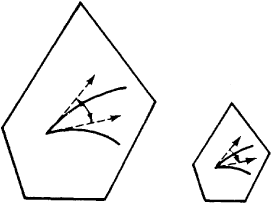Figure 1

that this conformal mapping is realized with the aid of the function of the complex variable z’ = z2. It is not difficult to ascertain that in this case the half-lines parallel to the sides of the angle are transformed into half-parabolas with a common focus at O’ (Figure 3). It should be noted that angles with the vertex at the point O increase by a factor of two; this does not contradict the definition of a conformal mapping, since O is not an interior point of the region.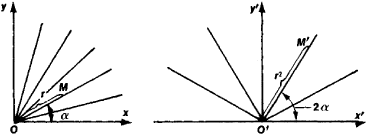Figure 2

In the general case of a conformal mapping, any curvilinear polygon P lying within the region being mapped is transformed into a curvilinear polygon P’ with the same respective angles, but the lengths of the sides may change in a nonproportional manner (Figure 4). However, if the polygon P shrinks to a point A, then its image P’ shrinks to a corresponding point A’ and the ratios of the lengths of the corresponding sides approach the same value:which depends only on the position of the point A (but not on the polygon being considered); it is called the contraction coefficient at the given point. This fact makes it possible to consider any conformal mapping “locally” (that is, in a sufficiently small neighborhood of each point A) as a similarity transformation followed, in general, by a rotation (see, for example, the quadrilaterals P and P’ in Figure 4).

Conformal mappings have long been used in cartography, when it has been necessary to depict part of a surface of the globe on a plane (a map) while preserving the magnitude of all the angles; examples of such conformal mappings are the stereographic and Mercator projections. The more general problem of the conformal mapping of an arbitrary surface (or part of it) to another surface (or part of it) is studied in differential geometry. Of special interest are the conformal mappings of certain plane regions to others; the theory of these mappings has vital applications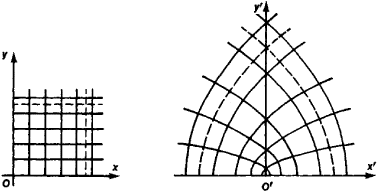Figure 3Figure 4

in hydromechanics, aeromechanics, electrostatics, and the theory of elasticity.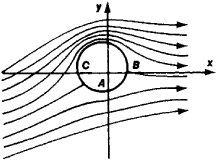Figure 5

The solution of many important problems can be easily obtained when the problems are posed for sufficiently simple regions (for example, circles or half-planes). If a problem is posed for another, more complex region, then we map conformally a simpler region onto the given one and obtain a solution of the complex problem from a known solution of the relatively simple problem. Thus, for example, the problem of determining the flow of an incompressible homogeneous fluid around a cylinder with a circular cross-section is relatively easy to solve. The lines of flow (that is, the lines along which the velocities of the fluid’s particles are directed) for this case are depicted in Figure 5; flow in the presence of circulation is given here. If the exterior of the circular cross-section of the cylinder is mapped conformally to the exterior of the cross-section of an aircraft wing (the wing profile), then, as can be shown, the flow lines for the case of the circular cylinder are transformed into the flow lines around the wing (Figure 6). Knowledge of the mapping function z’ = f(z) allows us to compute the flow velocity at any point, the lifting force of the aircraft wing, and so forth. N. E. Zhukovskii proceeded along these lines in creating the theory of the aircraft wing.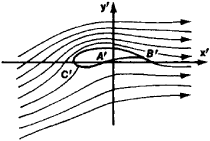Figure 6

Not all regions of the plane can be conformally mapped onto one another. Thus, for example, it is impossible to map conformally an annulus bounded by concentric circles of radii R1 and R2, where R1 < R2, onto another annulus bounded by circles of radii r1 and r2 where r1 < r2 if R2/R1 ≠ r2/r1. This makes it all the more remarkable that any two regions, each of which is bounded by only one curve (simply connected regions), can be mapped conformally onto each other (Riemann’s theorem). For example, any polygon can be mapped conformally onto any other polygon, as well as onto a half-plane or circle. Here the angles on the boundary, in general, change, but the definition of conformal mapping does not demand their preservation. As for regions bounded by several curves, such regions can always be mapped conformally onto regions bounded by the same number of mutually parallel line segments (Hilbert’s theorem) or circles (Koebe’s theorem). However, it is impossible to specify arbitrarily the sizes and mutual positions of these segments or circles.

A conformal mapping of one plane region onto another either preserves the sense of angles between curves—a conformal mapping of the first kind—or changes it into the opposite sense—a conformal mapping of the second kind. If a conformal mapping of the first kind is followed by a reflection in a line, then the result is a conformal mapping of the second kind (Figure 7).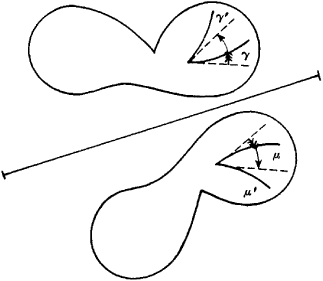Figure 7

If the complex variables z and z’ are introduced in the original and image planes, respectively, then z’, regarded as a function of z under the conformal mapping, is either an analytic function (conformal mapping of the first kind) or a function conjugate to an analytic function (conformal mapping of the second kind). Conversely, any function z’ = f(z) that is analytic in a given region and assumes different values [f(z1) ≠ f(z2) if z1 ≠z2] at different points of the region (such a function is called a schlicht function) maps the given region conformally to a certain region of the z’ plane. Therefore, the study of conformal mappings of plane regions reduces to the study of the properties of schlicht functions.

Every conformal mapping of three-dimensional regions transforms spheres and planes into spheres and planes and reduces either to a similarity transformation or to a product of an inversion and a similarity (Liouville’s theorem). Because of this, conformal mappings of three-dimensional (and in general higher-dimensional) regions are not as important nor do they have such diverse applications as conformal mappings of two-dimensional regions.

The foundations of the theory of conformal mapping were laid by L. Euler (1777), who established the importance of functions of a complex variable in the problem of the conformal mapping of a portion of a sphere onto a plane (the construction of geographical maps). The study of the general problem of the conformal mapping of one surface onto another led K. Gauss in 1822 to develop the general theory of surfaces. B. Riemann (1851) established the conditions under which it is possible to map one region (of the plane) conformally to another; however, the solution that he had outlined was successfully substantiated only in the early 20th century (in the works of H. Poincaré and C. Caratheodory). The investigations of N. E. Zhukovskii and S. A.. Chaplygin, who discovered a broad field of application for conformal mapping in aeromechanics and hydromechanics, served as a powerful stimulus for the development of the theory of conformal mappings as an important branch of the theory of analytic functions. In this field, the theoretical works of Soviet scientists are of major importance.

REFERENCES

Lavrent’ev, M. A., and B. V. Shabat. Metody teoriifunktsii kompleksnogo peremennogo, 3rd ed. Moscow, 1965.
Goluzin, G. M. Geometricheskaia teoriia funktsii kompleksnogo peremennogo, 2nd ed. Moscow, 1966.
Smirnov, V. I. Kurs vysshei matematiki, 8th ed., vol. 3, part 2. Moscow, 1969.
Markushevich, A. I. Teoriia analiticheskikh funktsii, 2nd ed., vol. 2. Moscow, 1968.
Koppenfels, W., and F. Stalmann. Praktika konformnykh otobrazhenii. Moscow, 1963. (Translated from German.)

A. I. MARKUSHEVICH

References in periodicals archive ?
Computation of conformal maps by modified Schwarz-Christoffel transformation," Ph.
We consider some conformal map projections in the common form as presented by Snyder (1987) as our reference basis.
Assume now that [LAMBDA] is a nontrivial conformal map.
By the very construction, [phi] is a conformal map which is the identity on R: thus it maps [R.
where [LAMBDA] is some suitable real linear conformal map, and then also quaternionic linear due to Lemma 6.
The characterization provided in  only depends on the chirality of the copy of H acting as codomain: moreover, for every choice of this chirality, all the conformal maps may be represented, independently of the sign of the determinant of their differentials.
Explicit formulas for conformal maps from the circular domains to the canonical slit domains [34, 35] for the multiply case case can be derived using the same techniques [13, 19].
In Section 2, we recall some useful preliminary facts about conformal maps and reflections in circles.
These functions could potentially be combined with conformal maps of circle domains [3,23] and Section 5.
The domain is the unit square [0,1] x [0,1]; the conformal map

Site: Follow: Share:
Open / Close